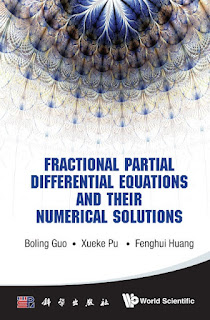DISCRETE MATHEMATICS

# Fractional Partial Differential Equations and Their Numerical Solutions PDF Free DownloadThis book aims to introduce some new trends and results on the study of the fractional differential equations, and to provide a good understanding of this field to beginners who are interested in this field, which is the authors’ beautiful hope. This book describes theoretical and numerical aspects of the fractional partial differential equations, including the authors’ researches in this field, such as the fractional Nonlinear Schrödinger equations, fractional Landau-Lifshitz equations and fractional Ginzburg-Landau equations. It also covers enough fundamental knowledge on the fractional derivatives and fractional integrals, and enough background of the fractional PDEs.